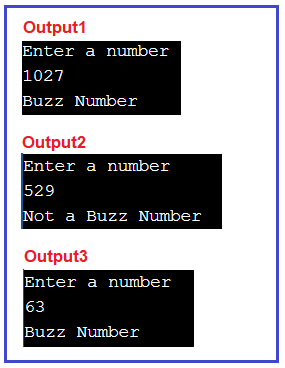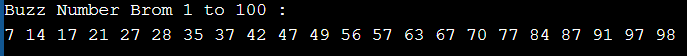# Buzz Number in C#

## Buzz Number Program in C# with Examples

In this article, I am going to discuss Buzz Number Program in C# with Examples. Please read our previous article where we discussed the Twisted Prime Number in C#. Here, in this article, first, we will learn what is a Buzz Number and then we will see how to implement the Buzz Number Program in C#. And finally, we will see how to print all the Buzz numbers between a range of numbers like between 1 to 100 or 100 to 1000, etc.

##### What is a Buzz Number?

A number is said to be Buzz Number if it ends with 7 OR is divisible by 7. The task is to check whether the given number is a buzz number or not. Or, for being a buzz number there are two conditions either of which must be true.

1. The number should end with digit 7 e.g., 27, 657, etc.
2. The number should be divisible by 7 e.g., 63, 49, etc.
###### Examples:

Input: 63
Output: Buzz Number
Explanation: 63 is divisible by 7, one of the conditions is satisfied.

Input: 72
Output: Not a Buzz Number
Explanation: 72 is neither divisible by 7 nor it ends with 7, so it is not a Buzz Number.

Input: 27
Output: Buzz Number
Explanation: 27 ends with 7, one of the conditions is satisfied.

To implement the Buzz Number, we are going to use the below two operators: –

Modulus %: Modulus Operator will calculate the remainder after an integer division.
Example: 97 % 10 = 7
43 % 10 = 3

Divides /: Divides numerator by de-numerator
Example: 97 / 10 = 9
43 / 10 = 4

##### How to Implement Buzz Number Program in C#?

We need to follow the below approach to implementing the Buzz Number Program in C#.

1. Input the number to check for the condition
2. Check whether the number is ending with digit 7 or divisible by 7
3. If the condition holds true print it’s a buzz number
4. If the condition doesn’t hold true print it’s not a buzz number
##### Example: Buzz Number in C#
```using System;
public class BuzzNumberProgram
{
public static void Main ()
{
Console.WriteLine ("Enter a number");
int number = Convert.ToInt32 (Console.ReadLine ());

if (number % 10 == 7 || number % 7 == 0)
Console.WriteLine ("Buzz Number");
else
Console.WriteLine ("Not a Buzz Number");
}
}
```
###### OutputTime Complexity: O(1) as there are atomic statements and no loops.

##### Buzz Number in between from 1 to 100

The following C# Program prints all the Buzz numbers between 1 to 100. As we know 1, 2, 3, 4, 5, and 6 are not Buzz Numbers, so, we started the for loop directly from 7.

```using System;
public class BuzzNumberProgram
{
public static void Main ()
{
Console.WriteLine ("Buzz Number Brom 1 to 100 :");
for (int number = 7; number <= 100; number++)
{
if (number % 10 == 7 || number % 7 == 0)
Console.Write (number + " ");
}
}
}
```
###### Output:In the next article, I am going to discuss How to Implement the Strong Number Program in C#. Here, in this article, I try to explain How to Implement the Buzz Number Program in C# with Examples and I hope you enjoy this Buzz Number Program in the C# article.